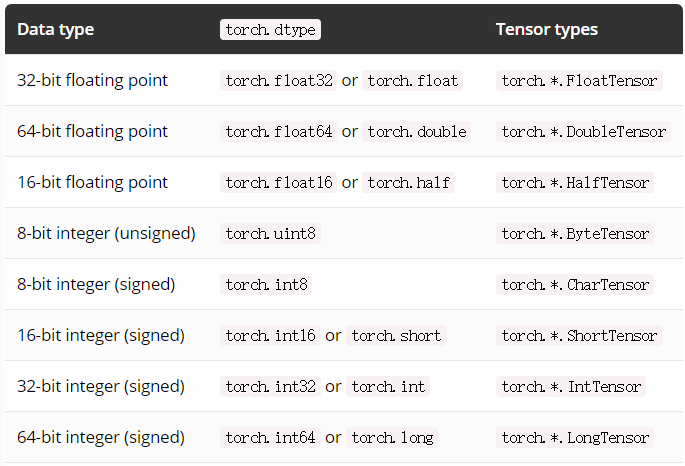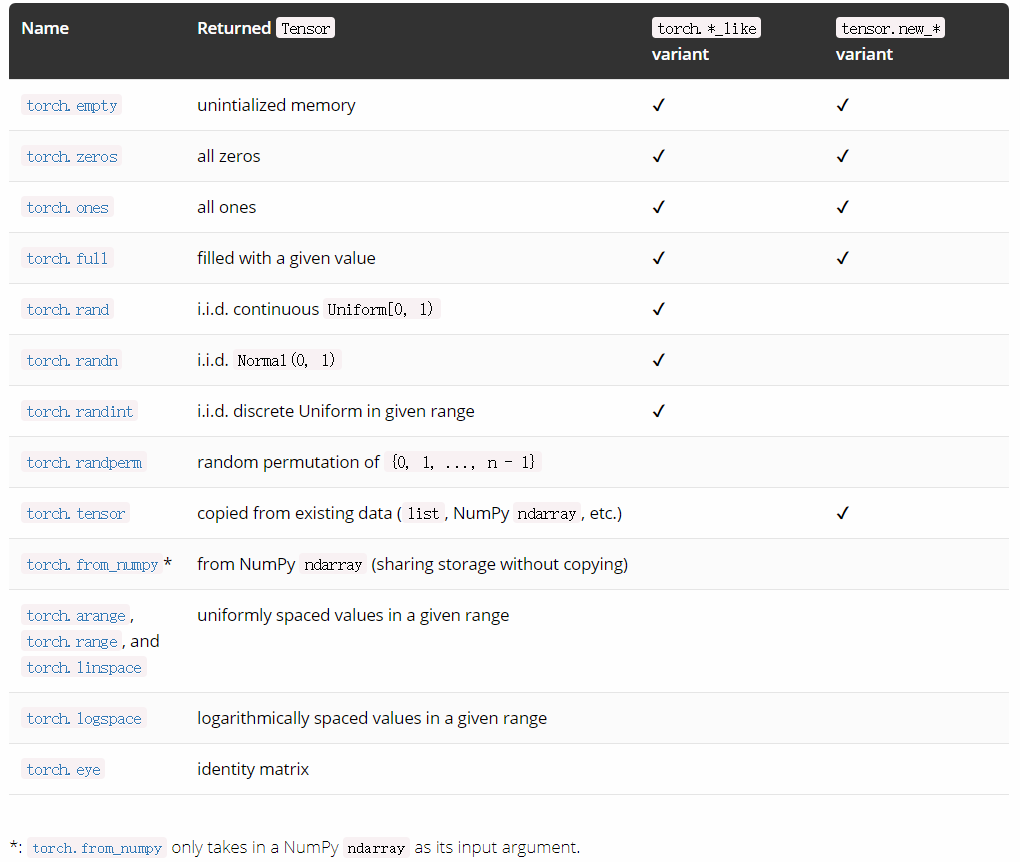# PyTorch 0.4 升级指南

4月25日，PyTorch团队正式发布了0.4.0的release版本。这是在与caffe2合并后的首个稳定版本。其中核心的变化有：

• Tensor/Variable合并
• 零维张量（标量）
• volatile标志的弃用
• dtypes,devices和Numpy型Tensor的创建函数
• 写设备无关的代码

# 合并Tensor与Variable类

torch.Tensortorch.autograd.Variable目前是同一类了。更确切地说，是torch.Tensor有了像Variable一样追踪历史与行为的能力。Variable像之前一样工作，但返回类型为torch.Tensor的对象。这意味着你不用再像之前在代码中到处使用Variable wrapper。

## type() 对Tensor作用发生改变

type()函数不再反映Tensor类的具体数据格式。可以使用isinstance() 或者x.type() 代替。

>>> x = torch.DoubleTensor([1, 1, 1])
>>> print(type(x))  # 原本会输出 torch.DoubleTensor
"<class 'torch.Tensor'>" # 但现在只会输出是一个torch.Tensor
>>> print(x.type())  # 正确: 'torch.DoubleTensor'
'torch.DoubleTensor'
>>> print(isinstance(x, torch.DoubleTensor))  # 正确: True
True

## 什么时候autograd开始追踪历史？

autograd的核心标志位：requires_grad，目前是Tensor的一个属性。之前应用于Variables的规则现在也适用于Tensors；因此autograd在当任何输入的Tensor操作的requires_grad=True时开始追踪历史。

>>> x = torch.ones(1)  # 创建一个requires_grad属性为False的Tensor (默认属性)
False # 默认为FALSE
>>> y = torch.ones(1)  # 另一个requires_grad属性为False的Tensor
>>> z = x + y
False
>>> # 接下来尝试验证追踪计算（反向传播）
>>> z.backward()
RuntimeError: element 0 of tensors does not require grad and does not have a grad_fn
>>>
>>> w = torch.ones(1, requires_grad=True)
True
>>> total = w + z
True
>>> # autograd 便可以计算其梯度
>>> total.backward()
tensor([ 1.])
True

### 对requires_grad标志位的操作

>>> existing_tensor.requires_grad_()
True
>>> my_tensor = torch.zeros(3, 4, requires_grad=True)
True


## 至于.data？

.data是从Variable中获得张量的方法。在这次合并后，调用y = x.data依然有相同的效果。y将是一个与x共享相同数据的Tensor，不包括x的历史，并且requires_grad=False

# 对0-维张量（标量）的支持

>>> torch.tensor(3.1416)         # 直接创建标量
tensor(3.1416)
>>> torch.tensor(3.1416).size()  # 标量是0维的
torch.Size([])
>>> torch.tensor().size()     # 与大小为1的矢量对比
torch.Size()
>>>
>>> vector = torch.arange(2, 6)  # 这是一个矢量
>>> vector
tensor([ 2.,  3.,  4.,  5.])
>>> vector.size()
torch.Size()
>>> vector                    # 通过标量对矢量进行索引
tensor(5.)
>>> vector.item()             # .item() 会以Python数的形式给出值
5.0
>>> mysum = torch.tensor([2, 3]).sum()
>>> mysum
tensor(5)
>>> mysum.size()
torch.Size([])

# volatile标志位的废除

volatile标志位现在已经废除并不会产生效果。之前任何涉及volatile=TrueVariable都不会被autograd所追踪。目前这已经被更灵活的上下午管理器如 torch.no_grad(), torch.set_grad_enabled(grad_mode),等所替代。

>>> x = torch.zeros(1, requires_grad=True)
...     y = x * 2
False
>>>
>>> is_train = False
...     y = x * 2
False
>>> torch.set_grad_enabled(True)  # 同样可作为函数使用
>>> y = x * 2
True
>>> y = x * 2
False

# dtypes,devices和Numpy型Tensor的创建函数

## torch.dtypeTensordtype可通过其dtype属性访问。

## torch.device

torch.device包含设备类型（cpucuda）和可选设备序号（id）。它可以通过torch.device（'{device_type}'）torch.device（'{device_type}：{device_ordinal}'）来初始化。

## torch.layout

torch.layout表示张量的数据分布。 目前支持torch.strided（稠密张量，默认值）和torch.sparse_coo（具有COO格式的稀疏张量）。

## 创建Tensor

>>> device = torch.device("cuda:1")
>>> x = torch.randn(3, 3, dtype=torch.float64, device=device)
tensor([[-0.6344,  0.8562, -1.2758],
[ 0.8414,  1.7962,  1.0589],
[-0.1369, -1.0462, -0.4373]], dtype=torch.float64, device='cuda:1')
>>> x.requires_grad  # default is False
False
>>> x = torch.zeros(3, requires_grad=True)
True

### torch.tensor(data, ...)

torch.tensor(data, ...)是新添加的张量创建方法之一。它采用各种类似数组的数据并将包含的值复制到新的张量中。 如前所述，torch.tensor是PyTorch中与NumPy的numpy.array等价的类型。 与torch.*Tensor方法不同，也可以通过这种方式（单个python数字在该对象中被视为Size 在torch.*Tensor方法中）创建0维张量（标量）。 此外，如果没有给出dtype参数，它会根据数据推断出合适的dtype。 这是从现有数据（如Python列表）创建张量的推荐方法。例如：

>>> cuda = torch.device("cuda")
>>> torch.tensor([, , ], dtype=torch.half, device=cuda)
tensor([[ 1],
[ 2],
[ 3]], device='cuda:0')
>>> torch.tensor(1)               # 标量
tensor(1)
>>> torch.tensor([1, 2.3]).dtype  # type inferece
torch.float32
>>> torch.tensor([1, 2]).dtype    # type inferece
torch.int64

1. torch.*_like除非另有说明，它默认返回一个与输入张量相同属性的张量：

>>> x = torch.randn(3, dtype=torch.float64)
>>> torch.zeros_like(x)
tensor([ 0.,  0.,  0.], dtype=torch.float64)
>>> torch.zeros_like(x, dtype=torch.int)
tensor([ 0,  0,  0], dtype=torch.int32)
2. tensor.new_*也可以创建与张量具有相同属性的张量，但它总是需要一个形状参数：

>>> x = torch.randn(3, dtype=torch.float64)
>>> x.new_ones(2)
tensor([ 1.,  1.], dtype=torch.float64)
>>> x.new_ones(4, dtype=torch.int)
tensor([ 1,  1,  1,  1], dtype=torch.int32)# 写设备无关的代码

PyTorch 0.4.0有两种更简单的方法：

• Tensordevice属性为所有张量提供了torch.deviceget_device仅适用于CUDA张量）
• Tensors和Modules的to方法可用于将对象轻松移动到不同的设备（而不必根据上下文调用cpu()或cuda()）

# at beginning of the script
device = torch.device("cuda:0" if torch.cuda.is_available() else "cpu")

...

# then whenever you get a new Tensor or Module
# this won't copy if they are already on the desired device
input = data.to(device)
model = MyModule(...).to(device)

# 代码样例

### 0.3.1

model = MyRNN()
if use_cuda:
model = model.cuda()

# train
total_loss = 0
for input, target in train_loader:
input, target = Variable(input), Variable(target)
hidden = Variable(torch.zeros(*h_shape))  # init hidden
if use_cuda:
input, target, hidden = input.cuda(), target.cuda(), hidden.cuda()
...  # get loss and optimize
total_loss += loss.data

# evaluate
for input, target in test_loader:
input = Variable(input, volatile=True)
if use_cuda:
...
...

### 0.4.0

# torch.device object used throughout this script
device = torch.device("cuda" if use_cuda else "cpu")

model = MyRNN().to(device)

# train
total_loss = 0
for input, target in train_loader:
input, target = input.to(device), target.to(device)
hidden = input.new_zeros(*h_shape)  # has the same device & dtype as input
...  # get loss and optimize
total_loss += loss.item()           # get Python number from 1-element Tensor

# evaluate
with torch.no_grad():                   # operations inside don't track history
for input, target in test_loader:
...`